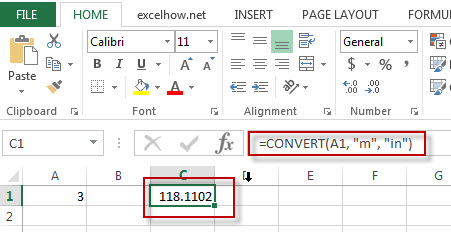# Excel Convert Function

This post will guide you how to use Excel CONVERT function with syntax and examples in Microsoft excel.

### Description

The Excel CONVERT function converts a number from one measurement system to another.

The CONVERT function is a build-in function in Microsoft Excel and it is categorized as an Engineering Function.

The CONVERT function is available in Excel 2016, Excel 2013, Excel 2010, Excel 2007, Excel 2003, Excel XP, Excel 2000, Excel 2011 for Mac.

### Syntax

The syntax of the CONVERT function is as below:

= CONVERT (number,from_unit,to_unit)

Where the CONVERT function argument is:
Number -This is a required argument. The value to be converted.
From_unit -This is a required argument. The units for number
To_unit -This is a required argument. The units for the result.

 Distance From_unit or to_unit Meter “m” Statute mile “mi” Nautical mile “Nmi” Inch “in” Foot “ft” Yard “yd” Angstrom “ang” Ell “ell” Light-year “ly” Parsec “parsec” or “pc” Pica (1/72 inch) “Picapt” or “Pica” Pica (1/6 inch) “pica” U.S survey mile (statute mile) “survey_mi”

The others Unit values can be referred to the official site https://support.office.com/en-us/article/CONVERT-function-d785bef1-808e-4aac-bdcd-666c810f9af2)

### Example

The below examples will show you how to use Excel CONVERT Function.

#1 =CONVERT(A1, “m”, “in”)Note: The above excel formulas will convert the value of B1 cell from “meter” unit to “Inch”.